## A fresh tricky TA dataset on weighted average

### GMAT/MBA Expert

GMAT Instructor
Posts: 645
Joined: 26 Jul 2010
Location: US
Thanked: 527 times
Followed by:227 members

### A fresh tricky TA dataset on weighted average

by e-GMAT » Sun Jan 20, 2013 11:22 pm
Try this tricky question fresh from the e-GMAT bakery!

The data given below for 15 movies on their performance on 3 attributes -Performance, Entertainment, and Script. Each attribute is awarded marks out of 10 by a select group, out of which Total Score Point is calculated.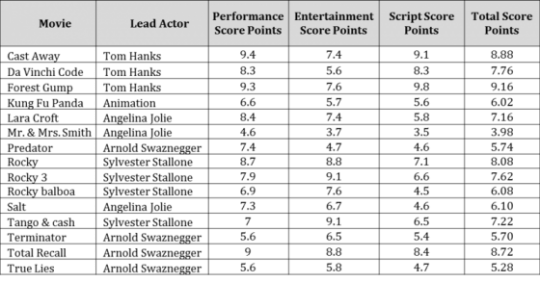For each of the following statements, select 'Yes' if statement is true based solely on the information provided in the table; otherwise select 'No'.

1. In the calculation of Total Score Points, equal weightage was given to both Performance Score Points and Script Score Points.
2. If the weightage of Entertainment Score Points is doubled to calculate Total Score Points, then there are exactly 9 movies, whose Total Score Points will increase.
3. If for each of the Arnold Swaznegger movies, respective movie Performance Score Point is swapped with Script Score Point, then the Total Score Point of exactly one movie of Arnold Swaznegger will decrease.

I have attached an excel file of this table. Please make sure that you only use sorting feature of Excel, that too in ascending order only.

Want to view similar table analysis questions with an interactive audio visual soltion? Register here at e-GMAT.

-Shalabh Jain
Attachments
TAble 1.xlsx
(10.42 KiB) Downloaded 46 times
Last edited by e-GMAT on Sun Jan 27, 2013 10:02 am, edited 4 times in total.

### GMAT/MBA Expert

GMAT Instructor
Posts: 645
Joined: 26 Jul 2010
Location: US
Thanked: 527 times
Followed by:227 members

### A fresh tricky TA dataset on weighted average

by e-GMAT » Wed Jan 23, 2013 8:26 am
e-GMAT wrote:Try this tricky question fresh from the e-GMAT bakery!

The data given below for 15 movies on their performance on 3 attributes -Performance, Entertainment, and Script. Each attribute is awarded marks out of 10 by a select group, out of which Total Score Point is calculated.For each of the following statements, select 'Yes' if statement is true based solely on the information provided in the table; otherwise select 'No'.

1. In the calculation of Total Score Points, equal weightage was given to both Performance Score Points and Script Score Points.
2. If the weightage of Entertainment Score Points is doubled to calculate Total Score Points, then there are exactly 9 movies, whose Total Score Points will increase.
3. If for each of the Arnold Swaznegger movies, respective movie Performance Score Point is swapped with Script Score Point, then the Total Score Point of exactly one movie of Arnold Swaznegger will decrease.

I have attached an excel file of this table. Please make sure that you only use sorting feature of Excel, that too in ascending order only.

Want to view similar table analysis questions with an interactive audio visual soltion? Register here at e-GMAT.

-Shalabh Jain
OA Q1- Yes, Q2- Yes, Q3- No.

Do attempt Q2. It is really tricky.

-Shalabh
Last edited by e-GMAT on Sun Jan 27, 2013 10:02 am, edited 1 time in total.

Senior | Next Rank: 100 Posts
Posts: 45
Joined: 06 Apr 2012
Thanked: 3 times
Followed by:2 members
GMAT Score:500
by BTG14 » Sat Jan 26, 2013 7:39 pm
Please give me the solutions for this question

### GMAT/MBA Expert

GMAT Instructor
Posts: 451
Joined: 21 Jan 2010
Location: New York City
Thanked: 188 times
Followed by:118 members
GMAT Score:770
by Tommy Wallach » Sat Jan 26, 2013 10:32 pm
Hey E-Gmat,

I know you post a lot of stuff on here, but as a caring citizen of the Beat the GMAT, I have to ask that you please make sure your material is written correctly, and that it presents a realistic take on the test. Your original question has numerous grammatical errors. "Weightage" is not actually a word, and it's used all over the place.

Beyond that, I don't think this question realistically mimics a real IR question. As far as I can see, the effort that would be necessary to solve this is way beyond what the real test would do. If I'm wrong, please post your solution/explanation here on the site (as opposed to linking off-site).

Thanks,

-t
Tommy Wallach, Company Expert
ManhattanGMAT

If you found this posting mega-helpful, feel free to thank and/or follow me!

### GMAT/MBA Expert

GMAT Instructor
Posts: 645
Joined: 26 Jul 2010
Location: US
Thanked: 527 times
Followed by:227 members
by e-GMAT » Sun Jan 27, 2013 10:28 am
Hi Tommy,

It's good to have caring citizens on BTG. We do post some fresh content for the benefit of the community. And I hope your comment '...you post a lot of stuff on here....' is a compliment. So thank you for the acknowledgement.

Let me address the questions you have raised:

"Weightage" is a very common term used in Asia. Folks in India, Singapore, and most of other countries use this term. You may check some dictionary links on this term.
1: https://www.merriam-webster.com/dictionary/weightage
2: https://dictionary.reference.com/browse/weightage
Furthermore, the meaning is abundantly clear from its usage in the question.

At e-GMAT, we listen to our customers and create content to help them succeed. Weighted Average is a very commonly tested concept on GMAT IR and a few of our customers requested extra practice on the same. This question was created in response to the same. OG13 Question 20 tests the same concepts as tested in this question. Now you may think that these questions (both official and e-GMAT) require calculations, but trust me, they can be solved by pure logic (especially parts 2 and 3, part 1 does require some calculation). I invite you to try it out or wait for me to post the solution.

Since you are a caring and responsible citizen of BTG, I would like to put forth a practice that in my opinion will benefit the community. Whenever, you have a doubt on any content that is posted by an expert, just send a pm to the expert with your doubt. That would allow the expert to clarify/change/correct the same without confusing the community. I am sure folks at BTG would recommend something similar. Do let me know if you need help to use the PM feature. I will be happy to create a help video.

Thank you,

Rajat Sadana

### GMAT/MBA Expert

GMAT Instructor
Posts: 645
Joined: 26 Jul 2010
Location: US
Thanked: 527 times
Followed by:227 members

### A fresh tricky TA dataset on weighted average

by e-GMAT » Wed Jan 30, 2013 12:56 am
Now is the time to provide solution to this question. Before you look at the solution, I do recommend that you first read my article on weighted average https://www.beatthegmat.com/mba/2012/12 ... -you-weigh. This will make sure, you absorb the concept, and its application better.

Question 1-

In the calculation of Total Score Points, equal weight-age was given to both Performance Score Points and Script Score Points.

Understand the question

3 attributes -Performance, Entertainment, and Script's score points are primary data, and Total score points is a derived data. It means that Total score points is derived out of a mathematical relationship among Performance, Entertainment, and Script score points. The first part of the question i.e. Q1, states that their relationship is governed by weights, or we can simply infer that Total score points is not a simple average of Performance, Entertainment, and Script score points.

Performance, Entertainment, and Script score points might have been mixed in some unknown proportion to derive the value of total score points.

Per the first part of question, Is Weight of Performance score points = Weight of Script score points ?

Traditional Approach

Step 1- Say Total weight = 1. Out of which, weight of Performance score points = weight of Script score points = x, & Weight of Entertainment score points = y. So x+y+x=1 => y=1-2x.

Step 2- Formulate mathematical formula

Total score points = x* Performance score points + y* Entertainment score points + x* Script score points

We can simplify this by plugging in the value of y as 1-2x. So the equation becomes Total score points = x*( Performance score points + Script score points )+ (1-2x)* Entertainment score points.

This gives,

Step 3- Pick any of the 2 listed movies, and plug in the relevant score points in the equation above. If value of x is same for both the movies, then we an say our assumption of taking weight of Performance score points = weight of Script score points was correct.

Say, we take Predator, & Total Recall movies...

For Predator, This gives x=0.4.

Now, we try with Total Recall, This also gives x=0.4.

This means our assumption of taking weight of Performance score points = weight of Script score points was correct. So the answer is Yes.

Well, I do not really recommend this approach, as this approach involves handling 2 variables x, & y.

Alternate Approach

Step 1- Say weight of Performance score points = weight of Script score points = 1, & weight of Entertainment score points = z. So total weight = 2+z.

Step 2- Formulate mathematical formula

(2+z)* Total score points = 1* Performance score points + z* Entertainment score points + 1* Script score points

This gives,

Step 3- Pick any of the 2 listed movies, and plug in the relevant score points in the equation above. If value of z is same for both the movies, then we an say our assumption of taking weight of Performance score points = weight of Script score points was correct.

Say, we take Predator, & Total Recall movies...

For Predator, This gives z=0.5.

Now, we try with Total Recall, This also gives z=0.5.

This means our assumption of taking weight of Performance score points = weight of Script score points was correct. So the answer is Yes.

This means that Performance, Entertainment, and Script score points are weighted in the ratio of 1:0.5:1 => 2:1:2 => 40%:20%:40% (In terms of %)

Question 2-

If the weight-age of Entertainment Score Points is doubled to calculate Total Score Points, then there are exactly 9 movies, whose Total Score Points will increase.

Understand the question

Presently, the weight-age of Entertainment Score Points is 20% out of 100%. Now it is doubled. It means that it becomes 40% now. Not to accommodate extra 20% (40-20), Performance, & Script score points will have to sacrifice 10% each. So the new weigh-age of 3 attribute would be 30%:40%:30%.

[size=18]Approach[/size]

Before answering this question, let us look at the concept of weighted average. If you have not visited my weighted article till now, pl. do so now.what-you-choose-is-what-you-weigh-143895.html

Say, there are 3 numbers 10, 12 & 20. Their average would be 14.
Now, a one more number 12 is added to it. So we have 4 #. 10, 12, 12 & 20.

Now, my question to you is- Can you deduce whether the average of 4 # would be less than 14 or more than 14?

Weighted Average Fundamental- If a new data, whose value is less current average of few data is added, then new average would be less than the current average. Similarly, vise-versa true for greater value.

Why is so?- It is because, the the new data pulls the average towards it.

Now, coming to our question- If the weight-age of Entertainment Score Points is doubled to calculate Total Score Points, then there are exactly 9 movies, whose Total Score Points will increase.

We are interested to know, new Total Score Points will increase compared to current Total Score Points or not.

So per Weighted Average Fundamental , Entertainment Score Points weight-age is doubled, or we can say that a new data is included. We can deduce that for those movies, whose Entertainment Score Points is more than Total Score Points, new Total Score Points will increase.

There are exactly 9 movies, whose Entertainment Score Points is more than Total Score Points. these are Lara Croft, Rocky, Rocky 3, Rocky Balboa, Salt, Tango & Cash, Terminator, Total Recall, True Lies. Answer is Yes.

Question 3-

If for each of the Arnold Swaznegger movies, respective movie Performance Score Point is swapped with Script Score Point, then the Total Score Point of exactly one movie of Arnold Swaznegger will decrease.

Understand the question

Currently, Performance, Entertainment, and Script score points are weighed in the ratio of 40%:20%:40%. The weights of Performance, and Script score points are equal, hence swapping will have no impact. Answer is N0.

Hope this explanation helps.

-Shalabh

### GMAT/MBA Expert

GMAT Instructor
Posts: 645
Joined: 26 Jul 2010
Location: US
Thanked: 527 times
Followed by:227 members
by e-GMAT » Wed Jan 30, 2013 1:04 am
Hi,

Some of you may not have an access to OG13. I have attached OG 13/#20 question for your ready reference. It tests weighted average concept.

-Shalabh
Attachments
OG 13-#20.docx
(26.69 KiB) Downloaded 49 times

### GMAT/MBA Expert

GMAT Instructor
Posts: 3380
Joined: 03 Mar 2008
Thanked: 2256 times
Followed by:1524 members
GMAT Score:800
by lunarpower » Wed Jan 30, 2013 10:17 am
hello,

first, tommy is correct: part 1 of this problem (and, by extension, part 3, which depends on the solution of part 1) requires far too much computation. this problem is well beyond the ken of even the quant problems, let alone the IR problems.

second, well... the given solution doesn't work.

viz.:

let's take the following 2 movies:
movie 1: performance 6, entertainment 7, script 9, total score 7.2
movie 2: performance 7, entertainment 8, script 10, total score 8.2

if you use "method 1", you'll get x = 0.2. this does represent one possible solution: if the weights are 20%, 60%, and 20%, you'll get those total scores.

but!
if the weights are 40%, 30%, and 30% -- in which "performance" and "script" are NOT equal -- you'll get the same total scores.
the given method isn't going to find this solution, because the given method just assumes (without justification) that those percentages are equal.

the "alternate approach" has the same problem. if you assume weights of 1, z, and 1, you'll get z = 3; this solution corresponds, again, to weights of 20%, 60%, and 20%.
again, though, this method won't find 40%, 30%, 30%, which is also a valid solution.

there are also other solutions for these numbers, too; e.g.,
30%, 45%, and 25% give the same total scores
10%, 75%, and 15% give the same total scores
and much more.

so, (a) the given solution method doesn't actually work, and, therefore, (b) the problem is even more complicated than it initially seems.
Ron has been teaching various standardized tests for 20 years.

--

Pueden hacerle preguntas a Ron en castellano
Potete chiedere domande a Ron in italiano
On peut poser des questions Ã  Ron en franÃ§ais
Voit esittÃ¤Ã¤ kysymyksiÃ¤ Ron:lle myÃ¶s suomeksi

--

Quand on se sent bien dans un vÃªtement, tout peut arriver. Un bon vÃªtement, c'est un passeport pour le bonheur.

Yves Saint-Laurent

--

Learn more about ron

### GMAT/MBA Expert

GMAT Instructor
Posts: 3380
Joined: 03 Mar 2008
Thanked: 2256 times
Followed by:1524 members
GMAT Score:800
by lunarpower » Wed Jan 30, 2013 10:43 am
at the risk of being overly forward, i thought i'd step into the following discussion, too:
e-GMAT wrote:"Weightage" is a very common term used in Asia. Folks in India, Singapore, and most of other countries use this term. You may check some dictionary links on this term.
1: https://www.merriam-webster.com/dictionary/weightage
2: https://dictionary.reference.com/browse/weightage
Furthermore, the meaning is abundantly clear from its usage in the question.
ok, but this test is not from one of those countries. it's an american test, and so uses american terminology. if a thoroughly educated native speaker of native american english (= tommy) states that "weightage" isn't used in the US (it isn't, by the way), he's trying to help!
there isn't really an argument here: if the US, and therefore the GMAT, wouldn't use a term, then the term shouldn't be used in prep materials, either.

also, the meaning is not "abundantly clear". nowhere does the problem actually state that the "total score" is actually a simple weighted average of the three components; the student is just expected to assume that it is one.
why not, say, a simple linear function? looking at the first and third points, for instance, they could both fit the formula 0.35(p + e + s) - 0.185 for total score. it's true that some of the other points wouldn't fit such a function, but the problem is still there: the student is expected to assume something that's not specified in the problem statement.
Whenever, you have a doubt on any content that is posted by an expert, just send a pm to the expert with your doubt. That would allow the expert to clarify/change/correct the same without confusing the community.
i can't speak for tommy, but, to me, this request seems to defeat the purpose of the forum environment.
an open forum, in my understanding, is a place specifically designed for public questions and answers, not private ones. (in fact, the original meaning of "forum" is "a public square, used, among other things, for open debate".) if someone takes issue with something that i post, i have no problem with having the resultant discussion on a public thread; that's why we're here in the first place.

finally, if i were a student, i would be rather insulted by this last statement, that simply discussing the merits of a problem could "confuse the community".
Ron has been teaching various standardized tests for 20 years.

--

Pueden hacerle preguntas a Ron en castellano
Potete chiedere domande a Ron in italiano
On peut poser des questions Ã  Ron en franÃ§ais
Voit esittÃ¤Ã¤ kysymyksiÃ¤ Ron:lle myÃ¶s suomeksi

--

Quand on se sent bien dans un vÃªtement, tout peut arriver. Un bon vÃªtement, c'est un passeport pour le bonheur.

Yves Saint-Laurent

--

Learn more about ron

Master | Next Rank: 500 Posts
Posts: 381
Joined: 19 May 2010
Thanked: 41 times
Followed by:2 members
by AbhiJ » Tue Feb 05, 2013 9:20 am
Ron,

To whoever in MGMAT who created the following IR problem,cannot help but comment on work ethic of the person. It must have taken lot of research to create this problem.

Standard Model of Particle Physics

https://gmatclub.com/forum/standard-mode ... 37529.html

PS: My post has no relation to e-GMAT discussion above. Just got reminded of this problem by the discussion.

### GMAT/MBA Expert

GMAT Instructor
Posts: 451
Joined: 21 Jan 2010
Location: New York City
Thanked: 188 times
Followed by:118 members
GMAT Score:770
by Tommy Wallach » Tue Feb 05, 2013 12:15 pm
Ron probably wrote it. He's a boss. : )

-t
Tommy Wallach, Company Expert
ManhattanGMAT

If you found this posting mega-helpful, feel free to thank and/or follow me!

### GMAT/MBA Expert

GMAT Instructor
Posts: 645
Joined: 26 Jul 2010
Location: US
Thanked: 527 times
Followed by:227 members

### A fresh tricky TA dataset on weighted average

by e-GMAT » Wed Feb 06, 2013 7:56 pm
Everyone,
Thank you for chiming in. In this response, I will address three things.
1. Whether this question is representative of an official IR question (to address the comment that the effort necessary to solve this question is beyond the scope of real test)?
2. Whether the solution provided is correct/applicable (to address the comment that this solution is incorrect)?
3. Why does the use case that Ron suggested not apply here (to address Ron's comments)
Please read below. I believe that we can give this discussion a rest at the end of this post.

IS THIS QUESTION REPRESENTATIVE OF AN OFFICIAL IR QUESTION?

e-GMAT's position: This question is based on an official IR question, and hence is very representative of an official question.

Explanation
This question is based on an official question - to be specific, OG13 - Q20. The official question is attached below. As you review both questions, you will find three striking similarities:
1.The datasets are similar - both questions have 3 entities and 3 weights.
2.The relationship between the columns (data entities) is very similar.
3.The question stem for first question is very similar to that of the official question.

a.OG question- "The score on the final exam had equal weight with the score on Exam 2 in computing the final score."
b.e-GMAT question- "In the calculation of Total Score Points, equal weightage was given to both Performance Score Points and Script Score Points."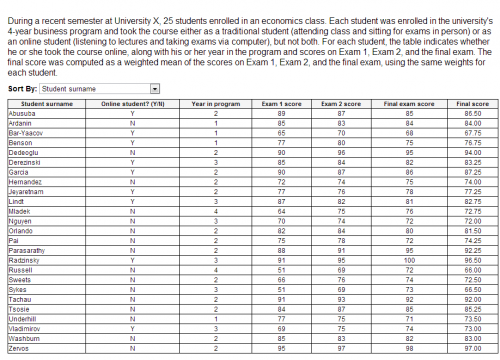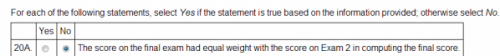As you can see, the e-GMAT dataset and first question are very similar to the corresponding elements of the official question and there is no point questioning whether an official question is computationally too intensive or not. It is an official question. It is there and we have to learn how to efficiently arrive at the answer.

WHETHER THIS SOLUTION IS CORRECT OR APPLICABLE??
Fundamental Principle: A set of "n" independent equations in "n" variables always leads to a unique solution set (i.e. a unique value for each of the three variables).
We have provided two approaches - referred to as "Traditional Approach" and "Alternate Approach" - both of which have been refuted in the post above. However, the traditional approach is the same as the official approach. .

Traditional Approach: The traditional approach is the same as that covered in the official solution. I have attached the screenshot of the official solution for reference. Labeling the Traditional Approach as incorrect will be the same as labeling the Official Solution incorrect. Here is the screenshot of the official solution.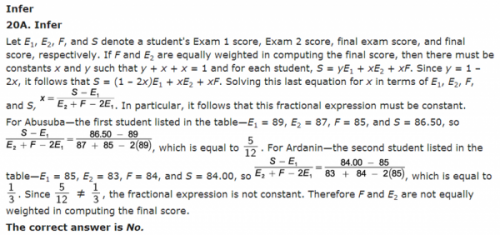This solution works because of the fundamental principle listed above. It uses three independent equations in three variables. Because of the principle cited above, the solution shall always be unique, enabling the test taker to choose a "definitive" Yes/No answer.

WHY DOES SUGGESTED USE CASE NOT APPLY HERE?

Here are 2 things that I want to bring to light about the suggested use case.

1.Rather than using the given dataset, the suggested use case was built using an arbitrary dataset - a dataset not provided by the question.

2.The arbitrary dataset was created based on a set of dependent equations, a scenario in which no definitive (Yes/No) answer exists. A question that "just" provides such equations is incorrect to begin with because there is no definitive answer. Note, the dataset provided in the e-GMAT question as well as in the official question eliminates such possibilities.

Read below to understand the points above.

Closer look at Suggested Use Case
In a weighted average question, there is one condition that is always true:
The sum of weights has to be equal to 1.
Hence, if x, y, z are three weights,

Weighted average condition: x+y+z = 1 - We will refer to this Eq3
Suggested Equation 1: 6x+7y+9z=7.2 - We will refer to this Eq1
Suggested Equation 2: 7x+8y+10z=8.2 - We will refer to this as Eq2

In the suggested use case it was proven that multiple values of x, y, z will satisfy these equations. However, there aren't two different equations; Eq1 and Eq2 are in fact the same equation. Read below:

Eq1:
6x + 7y + 9z = 7.2
6(x+y+z) + y + 3z = 7.2
Considering that x+y+z = 1 as per Eq3
EQ1 can be reduced to y+3z = 1.2
EQ1 can be reduced to y+3z = 1.2
Eq2:
7x + 8y + 10z = 8.2
7(x+y+z) + y + 3z = 8.2
Considering that x+y+z = 1 as per Eq3
EQ2 can be reduced to y+3z = 1.2, same as Eq1
EQ2 can be reduced to y+3z = 1.2
As proven above, Eq1 and Eq2 are really the exact same equation in the context of the question.

Hence, it's no surprise that there are multiple solutions. We need not even to do all this calculation. The fundamental principle tells us that if we try to calculate the values of 3 variables based on 2 equations, we will have infinite number of solutions, not just the 4 solutions as derived in the Suggested Use Case.

A question that just has such datasets is incorrect because it has not been framed properly to begin with as no definitive (Yes/No) answer exists. However, the official question and the e-GMAT question have been framed properly.

Now, could the solution be enhanced by adding a line instructing the student to ensure that the chosen dataset does not form dependent equations? Probably yes. However, categorizing a question, unrealistically complicated due to the required computation, especially one that is based on an official question, is probably not the best thing. Similarly, labeling the traditional approach incorrect especially when it's based on the official approach is incorrect as well.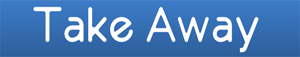1. The IR problem is very much similar to an official problem. Hence, labeling this question as "un-realistic" because of the amount of computation involved is same as labeling the official question as "un-realistic" - something that is not possible since official questions establish the reality.

2. The suggested Traditional Approach is also similar to the official approach. Suggesting that the traditional approach is wrong is suggesting that the official solution as wrong as well.
I am sure this post and our discussion so far contributes to the objectives of the forum i.e. to help students learn.

I respect the contributions that you have made on these forums and I appreciate your critical yet helpful feedback.

Thanks,
Rajat Sadana

### GMAT/MBA Expert

Site Admin
Posts: 6740
Joined: 13 Feb 2006
Location: Los Angeles, CA
Thanked: 1249 times
Followed by:988 members

### A fresh tricky TA dataset on weighted average

by beatthegmat » Wed Feb 06, 2013 8:10 pm
Thanks everyone for your participation on this thread. In reviewing the back and forth in this discussion, I'd like to remind our experts about our community rules, which can be found here: https://www.beatthegmat.com/mba/community-rules

In the Community Rules page, we have special rules for experts (GMAT instructors, MBA admissions consultants) at the bottom of the page. Please pay attention to the third bullet point, where we ask experts to avoid commenting on other competitor companies. We want to keep the spirit of the Beat The GMAT community friendly for all participants, and our experts in respectful terms with one another too.

Thanks for your help and thanks for all that you do to help our members achieve their dreams on the GMAT and MBA admissions!

(topic is now locked)
Beat The GMAT | The MBA Social Network
Community Management Team

Research Top GMAT Prep Courses:
https://www.beatthegmat.com/gmat-prep-courses

Research The World's Top MBA Programs:
https://www.beatthegmat.com/mba/school

• Page 1 of 1